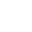# PHP Data Types

Variables can store data of different types, and different data types can do different things.

PHP supports the following data types:

• String
• Integer
• Float (floating point numbers – also called double)
• Boolean
• Array
• Object
• NULL
• Resource

# PHP String

A string is a sequence of characters, like “Hello world!”.

A string can be any text inside quotes. You can use single or double quotes:

Note: The output of above example will be two strings in one line. If you want these two lines in different line then use <br> HTML tag after first echo. Use the following example:

# PHP Integer

An integer is a whole number (without decimals).Â  It is a number between -2,147,483,648 and +2,147,483,647.

Rules for integers:

• An integer must have at least one digit (0-9)
• An integer cannot contain comma or blanks
• An integer must not have a decimal point
• An integer can be either positive or negative
• Integers can be specified in three formats: decimal (10-based), hexadecimal (16-based – prefixed with 0x) or octal (8-based – prefixed with 0)

In the following example \$x is an integer. The PHP var_dump() function returns the data type and value:

# PHP Float

A float (floating point number) is a number with a decimal point or a number in exponential form.

In the following example \$x is a float. The PHP var_dump() function returns the data type and value:

# PHP Boolean

A Boolean represents two possible states: TRUE or FALSE.

# PHP Array

An array stores multiple values in one single variable.

In the following example \$cars is an array. The PHP var_dump() function returns the data type and value:

Note: The output of the above code will be totally deserted. For better formating, use <pre> HTML tag which will better format the output of the array. Use the following code:

# PHP Object

An object is a data type which stores data and information on how to process that data.

In PHP, an object must be explicitly declared.

First we must declare a class of object. For this, we use the class keyword. A class is a structure that can contain properties and methods:

This is all about PHP Data Types. If you have any question, you can ask it at Forum Section.Automated page speed optimizations for fast site performance